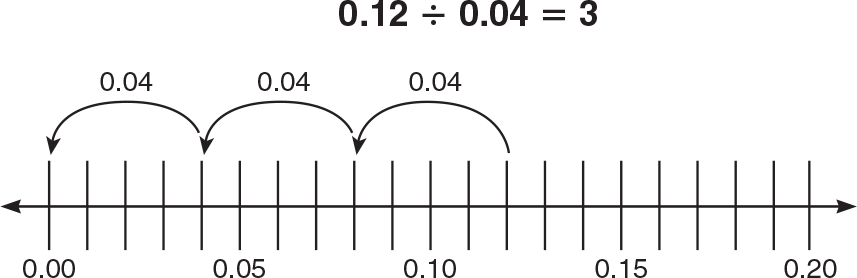1.800.221.5175
Core Program
Full Access
Core Program
Full Access
High-Impact Tutoring
Core Program
Full Access
National Program
Full Access
New York
Critical Thinking for Active Math Minds
Preparing for Standards Based Assessments
Vocabulary Workshop, Tools for Comprehension Grades 1–5
Print Program
Interactive Edition
Print Program
Interactive Edition
Vocabulary Workshop, Tools for Excellence Grades 6–12+
Print Program
Interactive Edition
Vocabulary for Success
Progress English Language Arts Grades K–8
National Program
Full Access
High-Impact Tutoring
New York
Grammar Workshop, Tools for Writing
Grammar for Writing
Print Program
Interactive Practice Bundle
Fluency Booster Practice Book
Interactive Assessments
High-Impact Tutoring
Building Reading Success with Wiley Blevins
Print Program
Interactive Edition
Professional Learning

## How Math Games and Activities Increase Student Proficiency in Grade 5

Math games and activities can be an excellent tool to supplement and support math instruction. Using math games in your classroom allow students to practice mathematics in fun but also impactful ways. Students love games because they are engaging and exciting, and teachers love games because they help students practice what they've learned.

In Grade 5, students can calculate the sums and differences of fractions and make reasonable estimates of them. Students can also multiply and divide fractions, explain numerical expressions, deepen their understanding of geometric principles, and develop strategies for problem solving. They will practice these skills using models, balancing equations, understanding proportions, and using rectangular arrays.

## Balancing an Equation

### Concrete representations are powerful tools to help students understand abstract concepts. This is why balance scales are an effective tool to help students understand the concept of equations and how to solve them. Without the distractions of letters and symbols, students see situations where two quantities are “in balance,” or equal to each other. Anything done to alter the makeup of one side must be done to both sides to maintain the balance.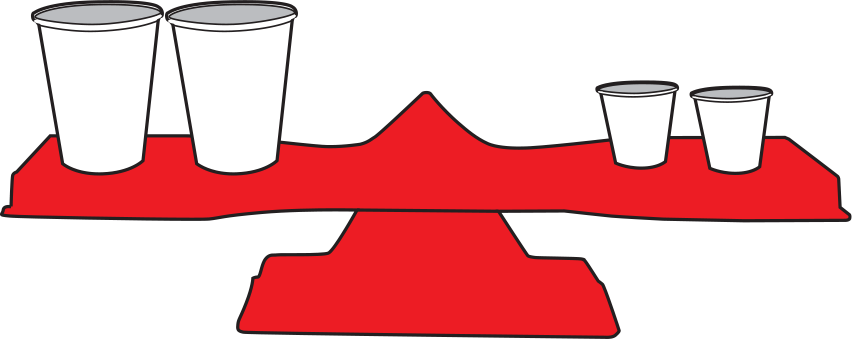Display a balance scale like the one above, and place cups of sand as shown. (The large cup must hold twice as much as a small cup.) Guide the discussion by asking questions such as: If you know the weight of a large cup of sand, can you find the weight of a small cup? If a large cup of sand weighs 8 ounces, what is the weight of a small cup?

Ask students to experiment with adding or removing large or small cups from this pan balance. If they remove a large cup of sand from the right side, the scale is out of balance. To maintain the balance, they must remove a large cup of sand from the left side. Removing one large cup from each side shows that one large cup is the same as 2 small cups. They can infer that a small cup weighs 4 ounces.

You can ask many different questions to reinforce students’ understanding during this process by varying the given information and by asking students to explain the strategies they used to solve.

By introducing the symbols used in an equation to represent the cups on the balance scale, you can transition into a more abstract representation.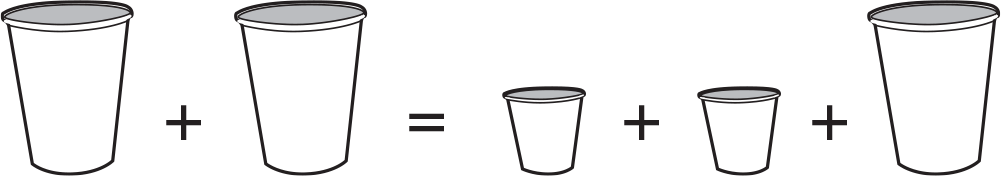The process is the same. Remove one large cup from each side.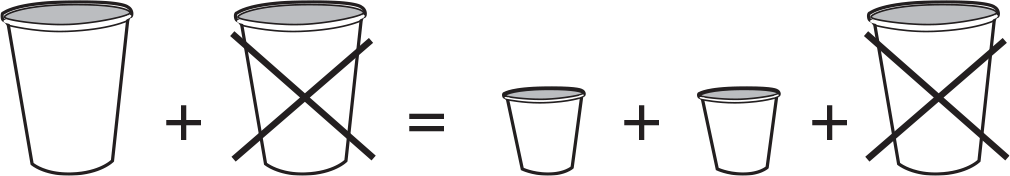Now you have: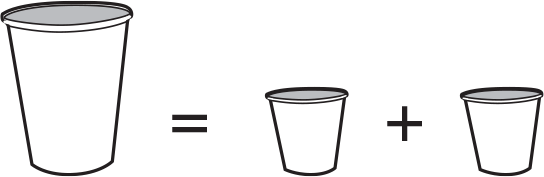If the small cup weighs 4 ounces, the weight of the large cup is 4 + 4, or 8 ounces. To bring the representation closer to formal algebra, you can replace the shapes with letters (variables) and have students solve again.

## Comparing Perimeter and Area

### Because students often confuse the perimeter and area formulas, they need many opportunities to apply and differentiate between them. Remind students that the perimeter of a polygon is the distance around the figure. The area of a figure is the number of square units that cover its surface. A square unit measures 1 unit on each side.

Below is an activity that can help students compare perimeter and area. Provide grid paper and ask students to outline all the possible rectangles with a perimeter of 36 units. Have students find the area of each rectangle, then organize the results in a table. Ask questions to help students reflect on the processes they use:

• What can you do to find the length and width of each rectangle? [Sample response: Since I know that P = 2l + 2w, I can find the different lengths and widths that equal 36 units.]
• How can you be sure you have found all the possible rectangles? [Sample response: I can systematically list all the lengths and widths that equal 36.]
• What must be the sum of 1l + 1w? Explain. [The sum must be 18, because 2l + 2w is 36 units.
• Are all areas equal? [No.] So figures with the same perimeter can have different areas. What do you notice about the shape of the figure with the greatest area? [It is a square.]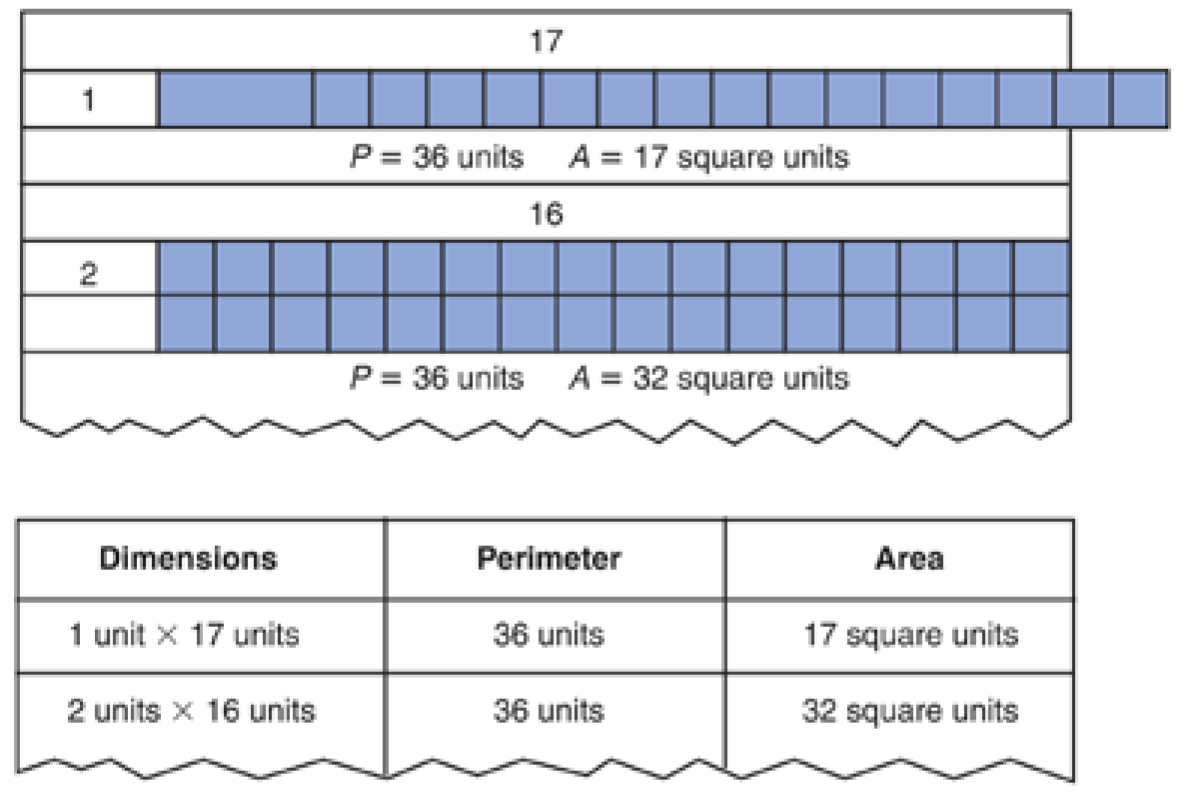To summarize, emphasize that the perimeter of complex figures stays the same no matter how the figure is separated into smaller figures. Guide students to draw their figures on grid paper and to count and then recount the lengths of the sides. Students may use a crayon to trace over each side to show that they have counted it. To extend the activity, have students use grid paper to make all the rectangles they can that have an area of 40 cm2. Ask them to share their methods for finding the solutions.

## Modeling Probability

### In fifth grade, students’ understanding of events that are equally likely and those that are more or less likely is basic to their acquisition of more formal skills and procedures in probability. Differentiating among these basic ideas can be carried out with an activity involving number cubes.

First, have students state the possible outcomes, and the probability of each, for tossing one number cube.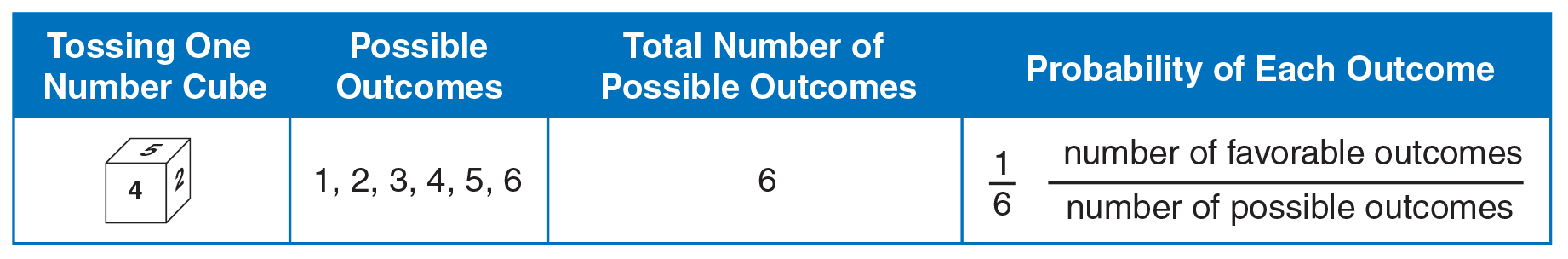Help students see that each outcome has an equally likely chance of occurring.

Next, add another cube to the activity. Have students state all possible sums when the cubes are tossed together. Students can list the sums in an addition chart.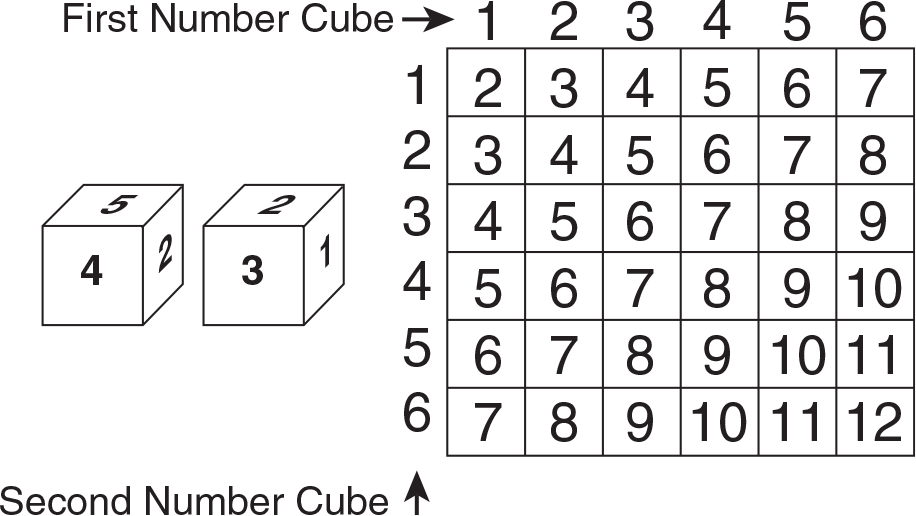Students can then count the number of favorable outcomes for each sum, the total number of possible outcomes, and record the results in a table or tally chart.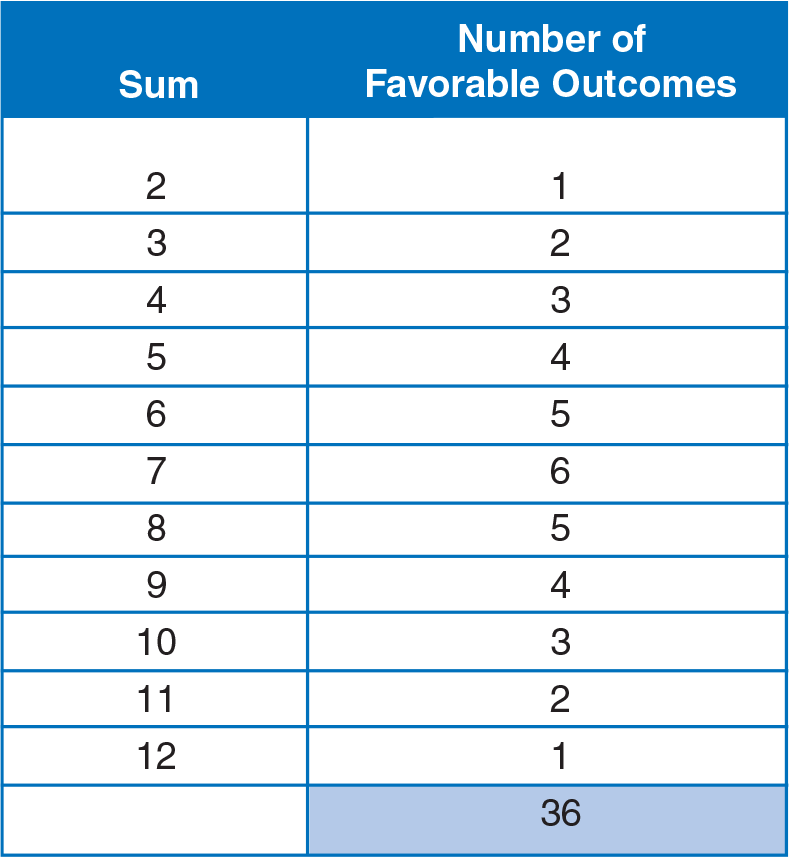Number of Possible Outcomes

Ask questions such as the following to guide students’ understanding:

• Does each sum have an equally likely probability of occurring?
• Which sum is most likely?
• Which sums are least likely?
• Is it more likely that a sum of 9 will occur or a sum of 7?
• Is it less likely that a sum of 12 will occur or a sum of 10?

If time permits, have students conduct an experiment where they actually toss two cubes 100 times, record the outcomes, and compare their actual results to what they expected would happen.

## Understanding Proportions

### Understanding ratio is a precursor skill for understanding proportion. Relating ratio concepts to previously learned fraction concepts ensures a smooth transition between the two areas. Lessons on ratio, equal ratios, and rates prepare students for concepts and applications of proportions.

Introducing proportions in the contextual framework of a calendar allows students to use what they already know about ratios to help them understand proportional relationships. Students know that there are 7 days in one week and can easily continue the following table.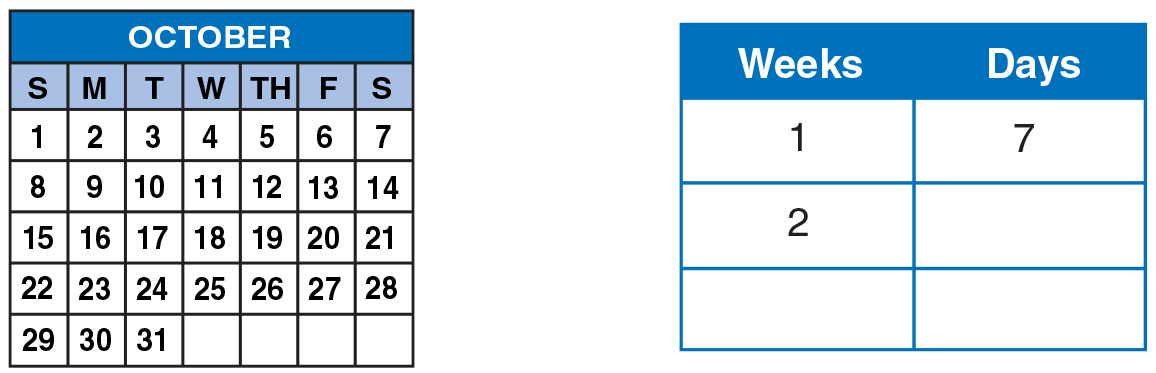The table emphasizes the multiplicative relationship between weeks and days. This relationship can also be expressed as different ratios in fraction form, for example 17 , 214 , 321 , and 428 .

Because a proportion uses different numbers while it maintains the same multiplicative relationship, a statement that any of these two ratios are equal is a proportion. In other words, the relationship of weeks to days is the same for all ratios in these proportions.

17 = 214 ; 17 = 321 ; 17 = n28 ; 17 = 5n

Each of these proportions is generated by an understanding of the primary relation-ship, 17 .

Students who have difficulty understanding the concept of a proportion as two equivalent ratios can use two-color counters to show the relationship of weeks to days. They might also recognize a pattern in the table and use this pattern to solve for the missing quantity.

Interpreting and working with ratios and proportions in this chapter prepares students for the new applications of percent they will encounter in later grades and daily life. Students’ grasp of ratio and proportion concepts is a prerequisite for understanding applications in probability and statistics, geometry, and algebra.

## Number Line

### A number line is an effective tool to help students visualize relationships between and among numbers.

The number line below is an example of how this tool can be used to find the least common multiple (LCM) of 4 and 5. The numbers at the top of the number line represent multiples of 4; the numbers at bottom of the number line represent multiples of 5. The number (20) where the lines meet for the first time is the least common multiple of 4 and 5.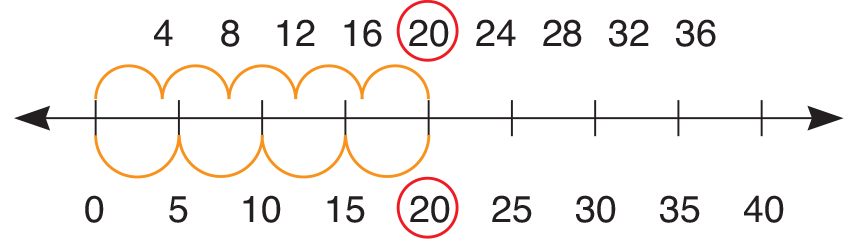Students who understand how to find the least common multiple will be able to understand the concept of finding the least common denominator (LCD) and will be prepared to add and subtract fractions with different denominators.

A number line can help students who have difficulty separating the rules for whole numbers from the rules for fractions. For example, some students think that 38 is greater than 12 because 3 is greater than 1 and 8 is greater than 2.1 The number lines below not only demonstrate that fractions can be thought of as numbers between whole numbers, but show that 12 is indeed greater than 38 .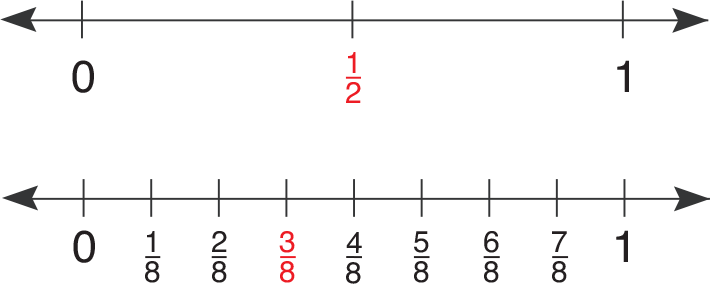The same pair of number lines can also be used to demonstrate that 12 and 48 are equivalent fractions.

Students who understand the relative magnitude of fractions will be able to easily compare and order sets of fractions with the same or different denominators.

1 Behr, Merlyn J., et al. March 1985. “Construct a Sum: A Measure of Children’s Understanding of Fraction Size.” Journal for Research in Mathematics Education, vol. 16, no 2: 120-131

## Base-Ten Models

to Divide

### At this level, base-ten models can be used to refresh students’ understanding of the meaning of division. Models can also help students connect division of a multi-digit dividend by a one-digit number to the algorithm.

Have students begin with a dividend and divisor that will result in a quotient with no remainder.

Use hundreds, tens, and ones to model  6 192

Display the following:Because the goal is to form 6 equal groups, students should recognize that they must first divide the hundreds. Equal groups cannot be formed until the hundred flat is traded for 10 ten rods.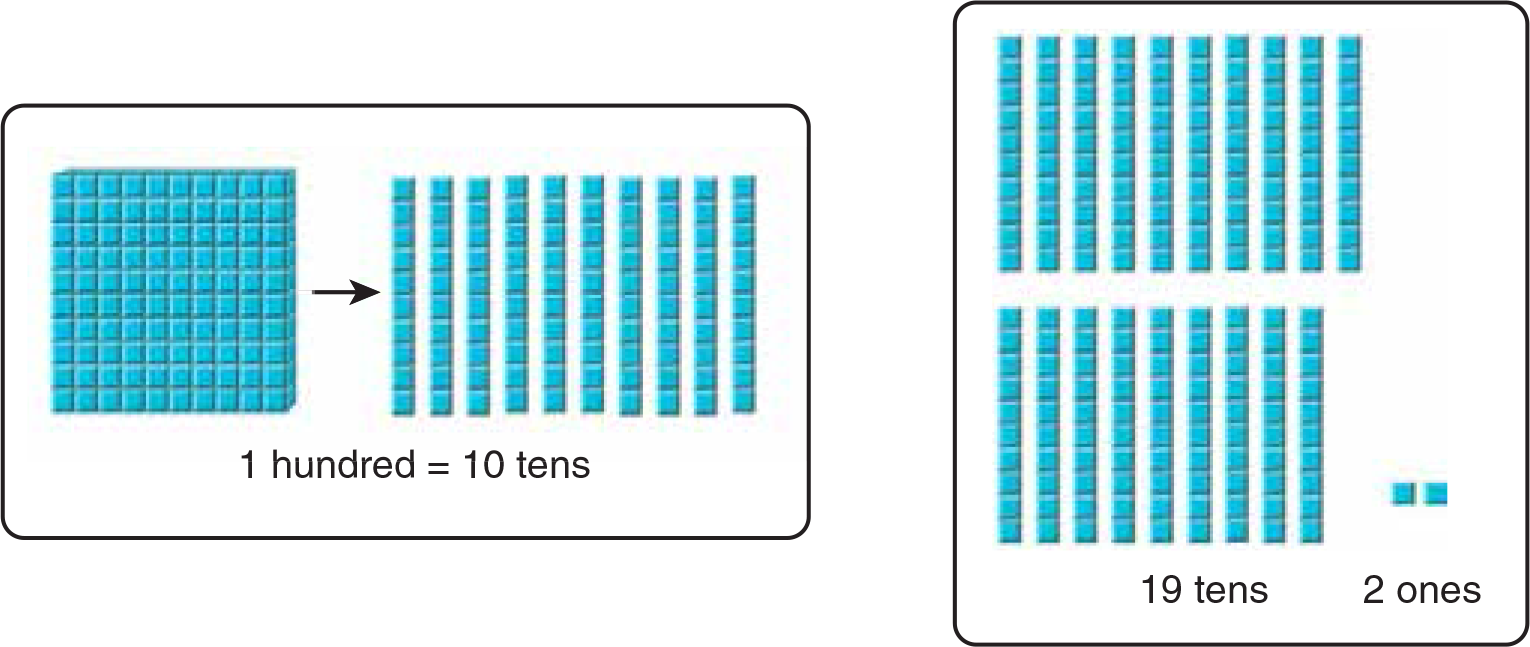Now the 19 tens can be equally partitioned into 6 equal groups. One ten will be left over.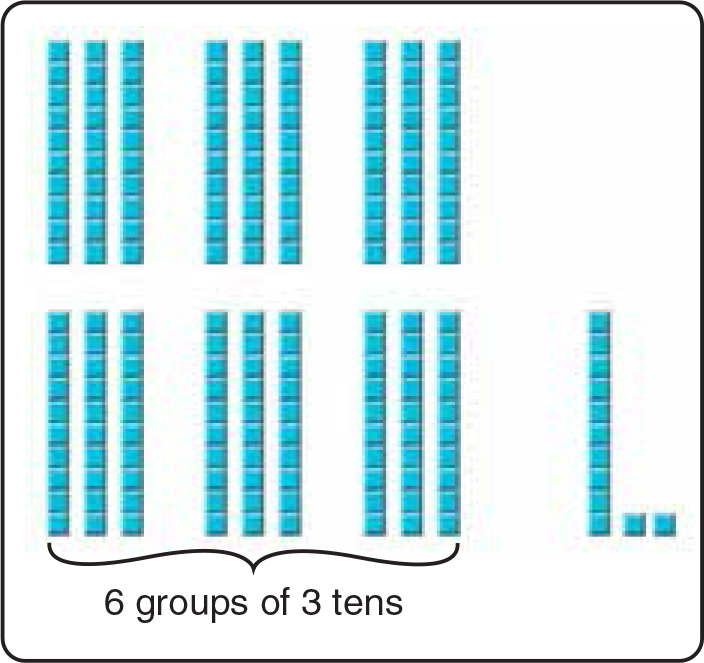The next step is to divide each ten into ones. One ten is traded for 10 ones. Then the 12 ones are equally shared among the 6 equal groups of 3 tens.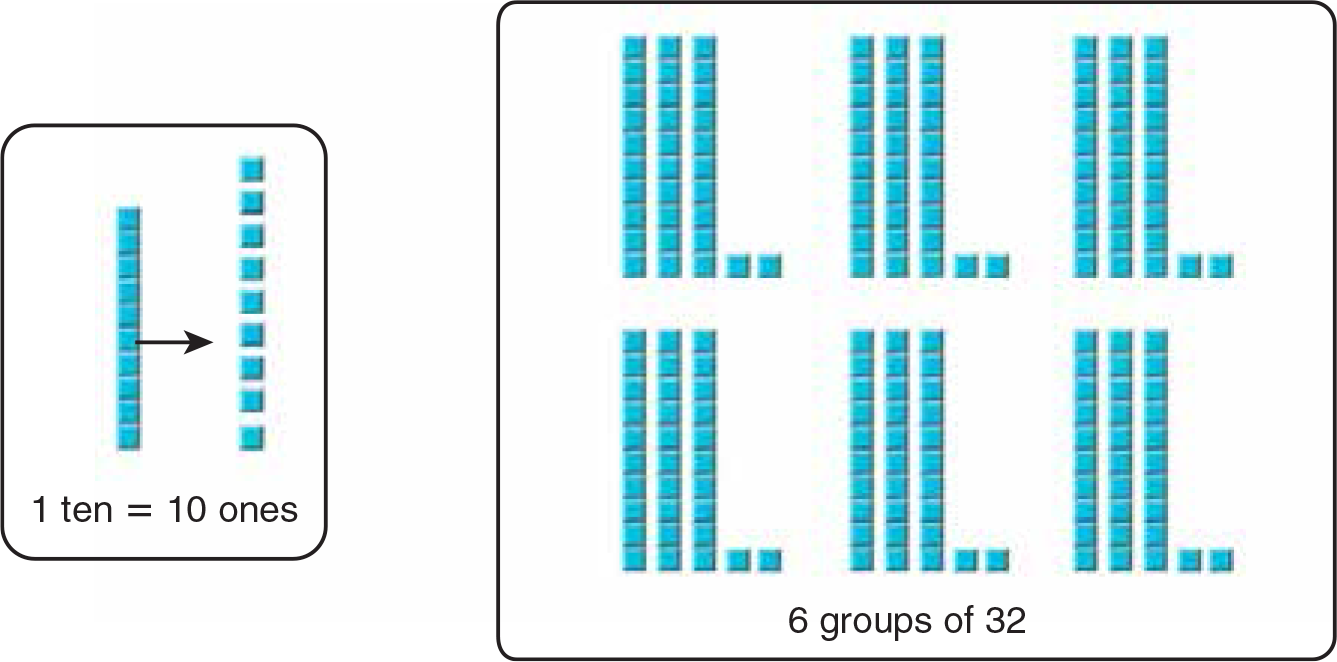It is important that students make the connections between the modeling activity and the symbolic form of the algorithm.

• Students should first summarize the steps used to model the division: Decide if hundreds can be divided. If not, trade hundreds for tens. Divide tens. Trade tens for ones. Divide ones. The quotient is the number in each group, 32.
• Then have students record the steps symbolically in the division house, labeling each step of the division.

Once this three-phase process is completed for division without remainders, students can use the same process to model division with remainders.

References
Van de Walle, J. A. (1998). Elementary and Middle School Mathematics: Teaching Developmentally, 55, 401 New York, NY

## Centimeter Grid Paper

### A simple to make, and easy to understand, decimal model can be constructed from centimeter grid paper, or if smaller models are preferred, graph paper. The models can then be shaded, colored, or cut apart to show addition and subtraction.

The first step is to create a representation of 1 as a referent. Students outline a 10 × 10 square and cut it out. Have them turn over the square to the blank side and write the decimal equivalents of 1 in tenths and hundredths: 1 = 1.0, or 1.00. Have students recall that each small square or unit represents 0.01, or 1100 . A column or row of 10 units represents 0.1, or 110 .

Next, students cut the grid into two pieces and write an addition equation for that representation. Students may choose to add benchmark numbers, for example 0.40 + 0.60 = 1.00, 0.50 + 0.50 = 1.00, or non-benchmark numbers, such as 0.37 + 0.63 = 1.00.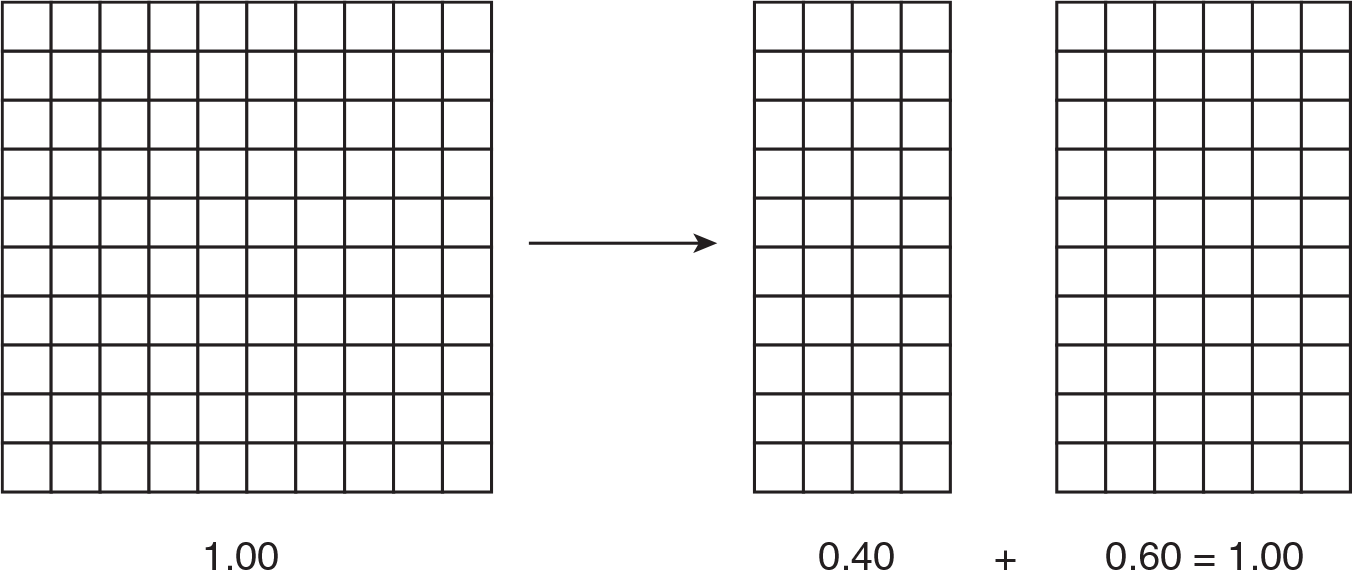You may wish to have students use color to designate the two decimals that compose and decompose 1.00 rather than have them cut the grids.

Working with hundredths, students need to compose or put the parts of a number together (or add), and decompose or separate a number into parts (or subtract) many times. Encourage students to show a variety of ways to represent their additions and subtractions to and from 1.00 before they begin working with thousandths.

After multiple experiences with 1.00, students can carry out similar composition and decomposition with decimals greater than (or less than) 1.00, such as 1.25 − 0.35.

To start, students create a model for 1.25 by outlining a 10 × 10 square to represent 1, 2 tenths columns to represent 0.2, and 5 hundredths squares to represent 0.05 on the grid paper. Color the hundredths red, and cross them out to show subtraction of the hundredths. Color 3 tenths columns yellow to show subtraction of the tenths. But before they color, help students recognize that to subtract the 3 tenths, they “regroup” 1 as 10 tenths. Then, students color the tenths and cross out to show the subtraction. Students count the remaining tenths to find the difference.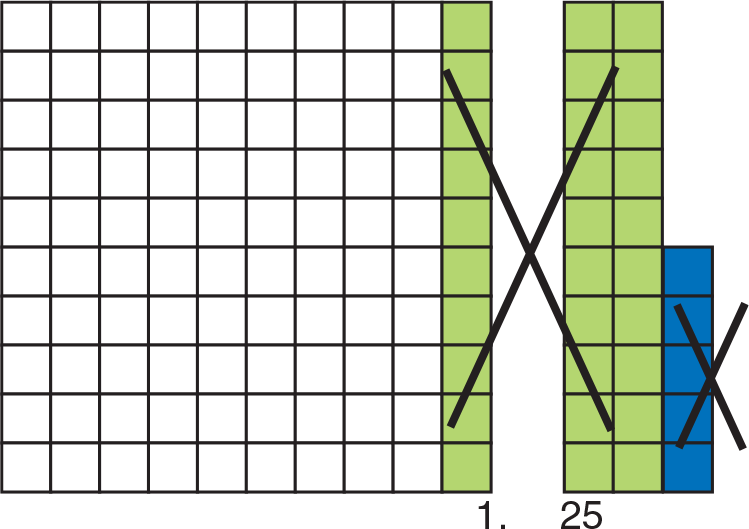## Fraction Strips

### Fractions can be added or subtracted only when the denominators are the same. To add or subtract fractions with unlike denominators, one or more of the fractions must be renamed as equivalent fractions with denominators.

For the following activity, you may wish to have students prepare sets of strips.

Have students find the sum of 34 + 56 , according to the rest of the example.

First, display the strip for each fraction.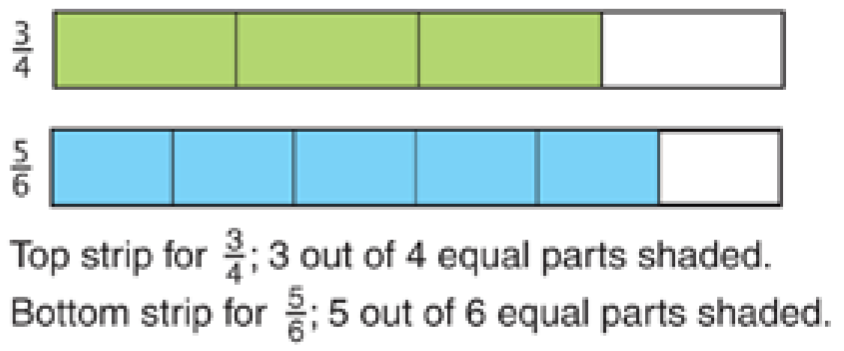Identify the strip that represents a common denominator of the two fractions. Guide students to use reasoning to decide that the common denominator is 12, so they should use the strip for twelfths. Have students model as shown.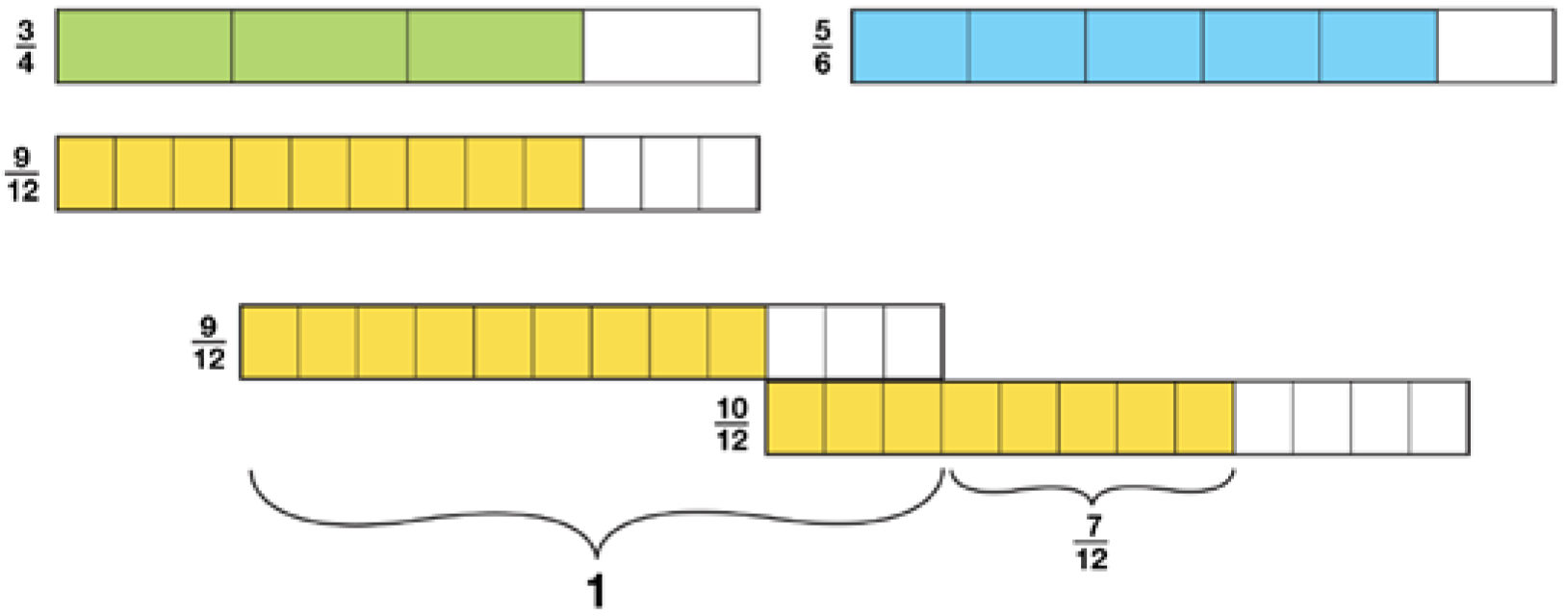They can record symbolically as they model or they can summarize: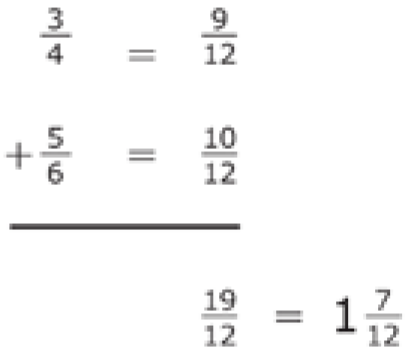To show that the same principle of renaming applies for subtraction, have students find the difference of 2312 . Point out that when they use fraction strips to subtract, they match the number of equal parts in the subtrahend to the number in the minuend, and the difference is the unmatched part.

Display the strip for each fraction.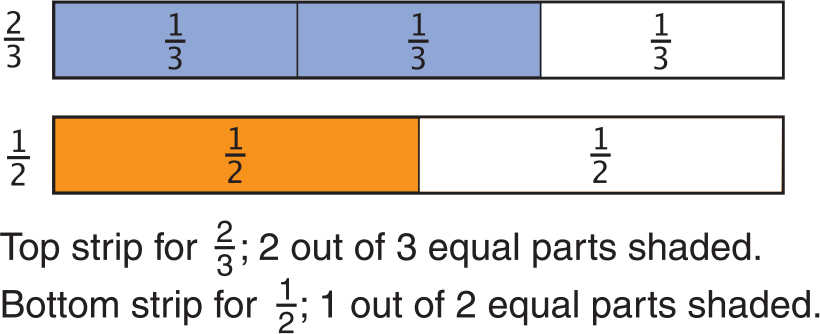Together, look for the strip that represents a common denominator of the two fractions.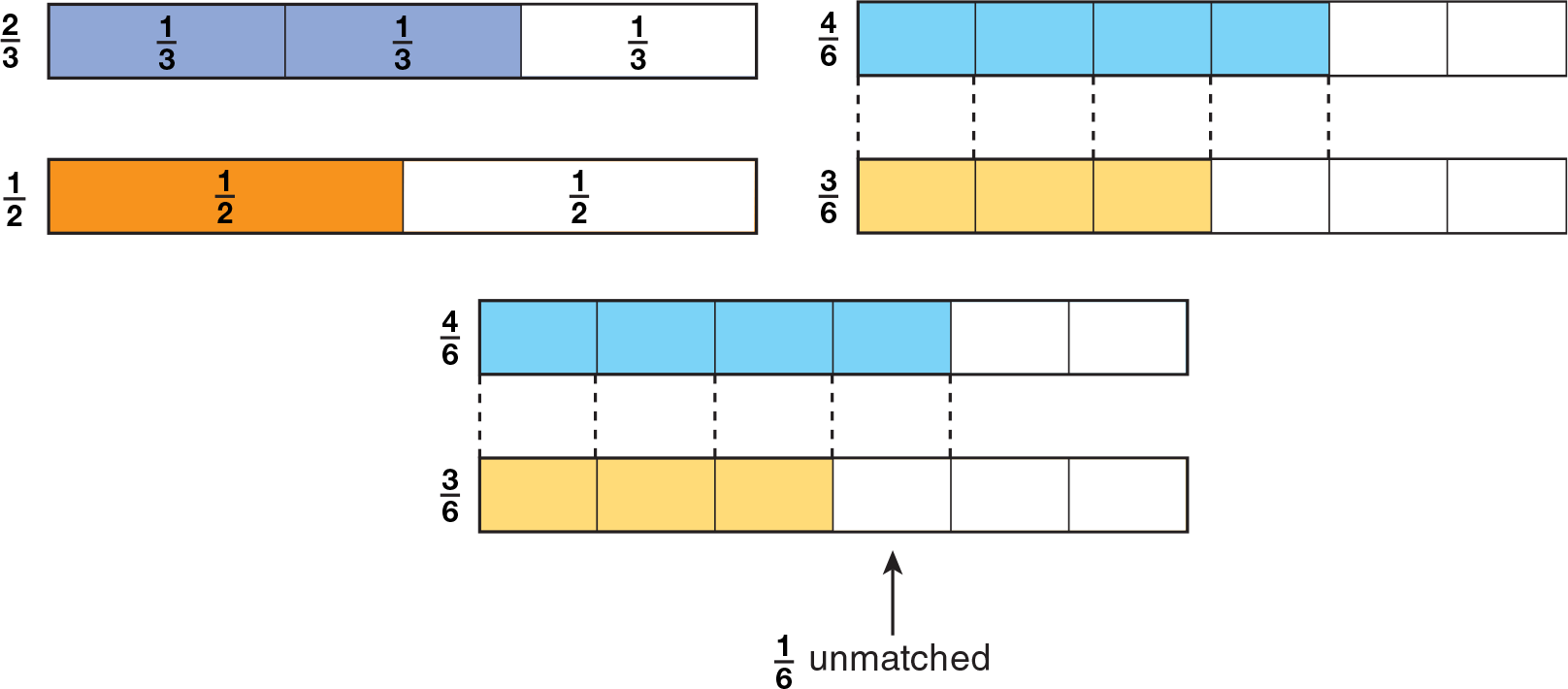Activities such as the above will help students understand why it is necessary to find the common denominator before adding or subtracting fractions with different denominators.

## Models to Divide Fractions

### Often, when students learn to divide with fractions, they simply memorize the steps “invert and multiply” without comprehending the value of this procedure. And although they also learn that two numbers with a product of 1 are reciprocals, or multiplicative inverses, this too is frequently not understood. You can provide students with opportunities to build insight by using patterning and models, as well as students’ prior knowledge of whole number division.

First, present a pattern that shows students how multiplication gives meaning to the term “reciprocal.”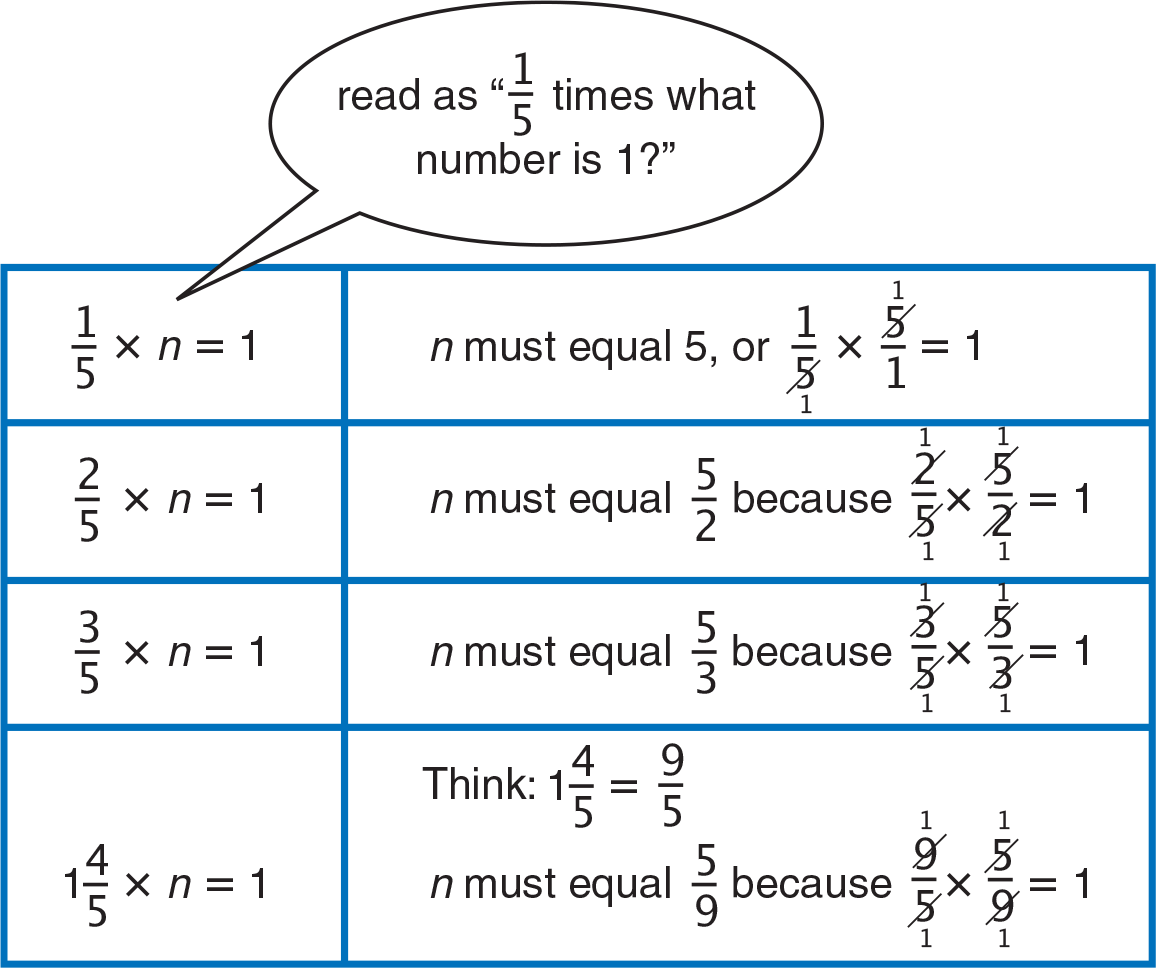Next, link division to finding a missing factor. For example, have students recall that for 63 ÷ 9 = n, they can think, “9 times what number is 63?” Point out that this can be expressed symbolically as 9 × n = 63. Now help students recognize the same relationship in a fraction problem:

Guide students to conclude that the numerator of n must be 1, and the denominator must be 2 because 4 × 2 = 8, in which case the missing factor is 12. Using the check step— 14 × 12 = 18—and relating it to 18 × 41 = 12 , students will begin to see why the “invert and multiply” step works.

Finally, build on students’ prior knowledge of whole number division by extending the meaning of division to fractions. Use a unit square to model 810 ÷ 210 = n. Students should express this as, “How many 210's are in 810 ?"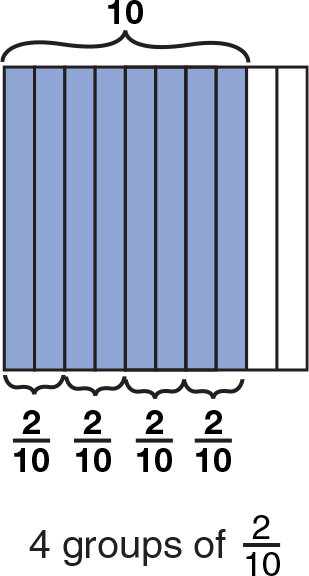## Paper Figures

to Find the Sum of the Angles

### Using paper to cut and combine the angles of plane geometric figures is a highly visual, hands-on way for students to explore and find the sum of the interior angles of triangles and quadrilaterals. You can use 3 × 5 or 4 × 6 index cards for this experiment involving the angles of a triangle.

Students cut a triangle from their index cards. They label the interior points of the angles with letters.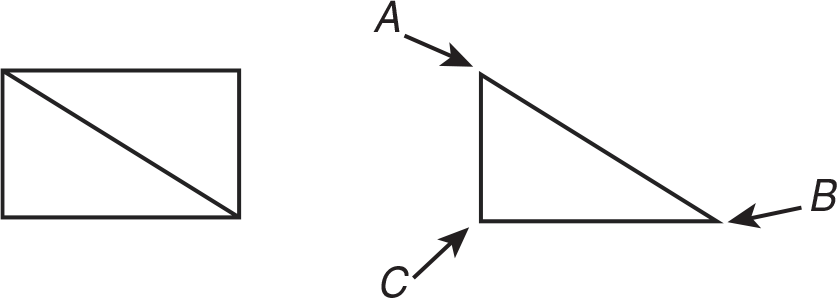Guide students to tear, not cut, the three angles off their triangles.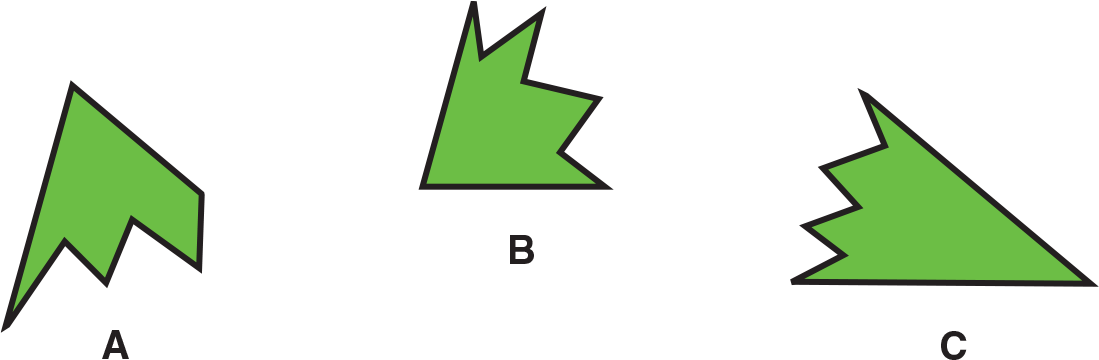Using a piece of notebook paper as a straight angle, students align the three smooth, pointed angles on an edge to form a straight line. Have them confirm that the sum of the measures of the three angles of a triangle always equals 180°.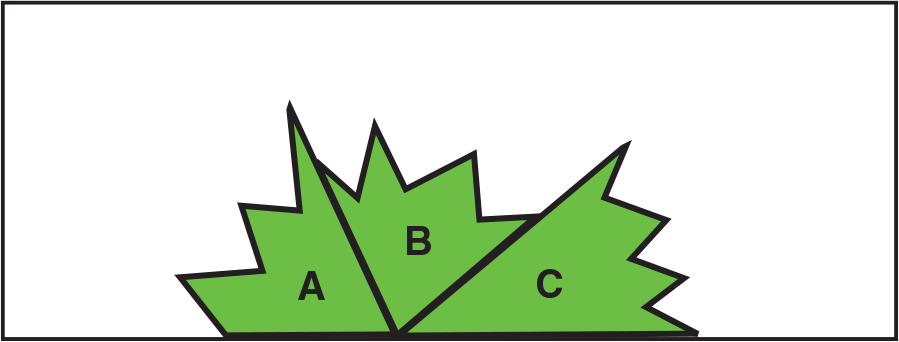Students should use their index card to repeat this procedure with different types and sizes of triangles. This provides multiple representations of the constant (180°) they are exploring. In addition it lays a foundation for determining the sum of angles of other figures.

This activity may also be used for confirming that the sum of the four angles of every quadrilateral is always 360°. Students label and then tear the angles off any quadrilateral. They place the angles in two groups as shown below to form two straight angles. Students should recognize that 2 × 180 = 360, so the four angles together equal 360°.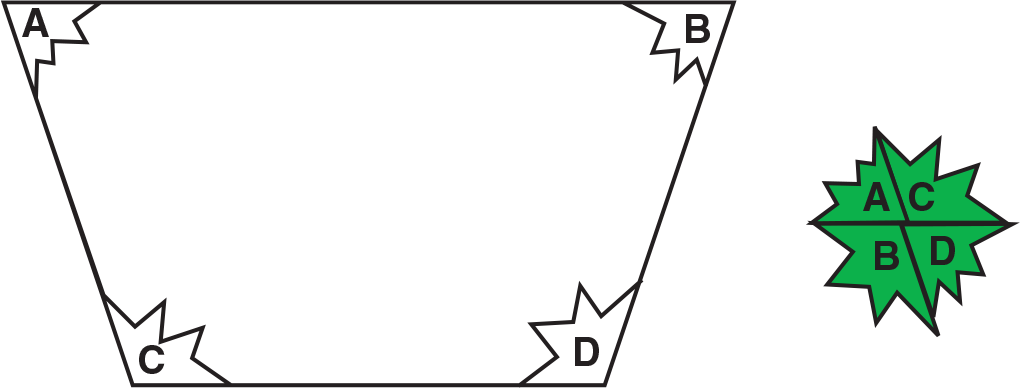## Place Value Models

### Base-ten blocks are clear, unambiguous representations of how our decimal number system builds each place to the left.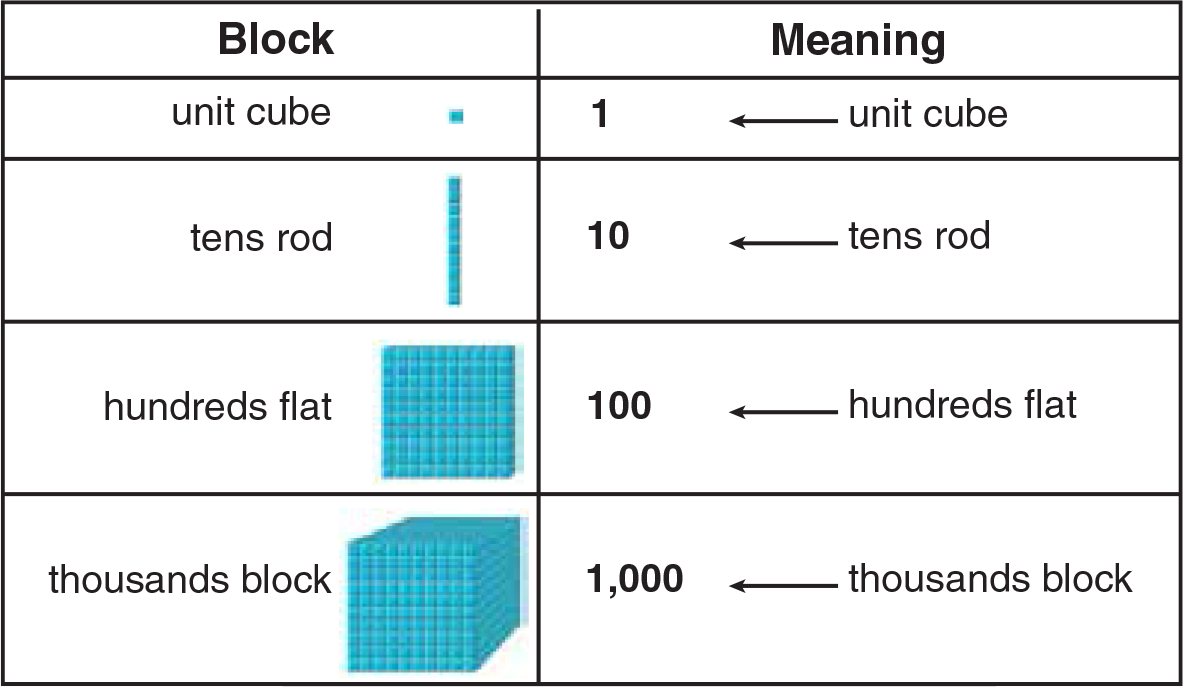To extend the above table beyond the thousands place, use the concept of grouping by tens to help students form a mental picture of each succeeding place. Work through the table first, by having students tell you how many unit cubes are needed to build a tens rod. A student volunteer can group ten unit cubes to form a tens rod or may draw a diagram to demonstrate. Then ask how many tens rods are needed to make up a hundreds flat. Let the volunteer continue to demonstrate either with tens rods or with diagrams. Continue with the demonstration for building a thousands cube by combining ten hundreds flats. For the next numbers in the sequence, have students mentally picture what each model would look like. Using and extending the sequence of cube, rod, flat, cube, and so on, students may recognize that ten thousand can be represented as a rod consisting of 10 thousands blocks; one hundred thousand can be represented as a flat consisting of 10 ten thousands rods, and so on.

Through verbalization and diagramming, students reinforce the repeating pattern that corresponds to periods in a number.To model a number, children should identify the digit in the tens place and lay out that many tens rods. They identify the digit in the ones place and lay out that many ones cubes.

To identify a number represented by a model, children count the number of tens rods to find the digit in the tens place. The number of ones cubes shows the digit in the ones place. Children can also count the total number of ones cubes in the entire model, but this brute force method is time consuming and ignores the merits of place-value concepts.

References
Van de Walle, J. A. (1998). Elementary and Middle School Mathematics: Teaching Developmentally, 55, 401 New York, NY

## Rectangular Arrays

in Multiplication

### Rectangular arrays and diagrams help students break apart multi-digit multiplication into—for example—hundreds, tens, and ones. Guide students to connect the models with the meaning of each part of the multiplication process. This will help them avoid mechanical errors such as recording digits in the wrong column of a partial product or forgetting to add a regrouped digit.

Students can use groups of base-ten blocks arranged in arrays to represent the partial products in a multiplication problem. Alternatively, students can use an area diagram to represent the partial products. The illustrations below show how both methods can be used to model 15 x 35. For both approaches, students should begin by breaking apart the expression, using the Distributive Property of Multiplication Over Addition.Next, model with base-ten blocks, and record the findings: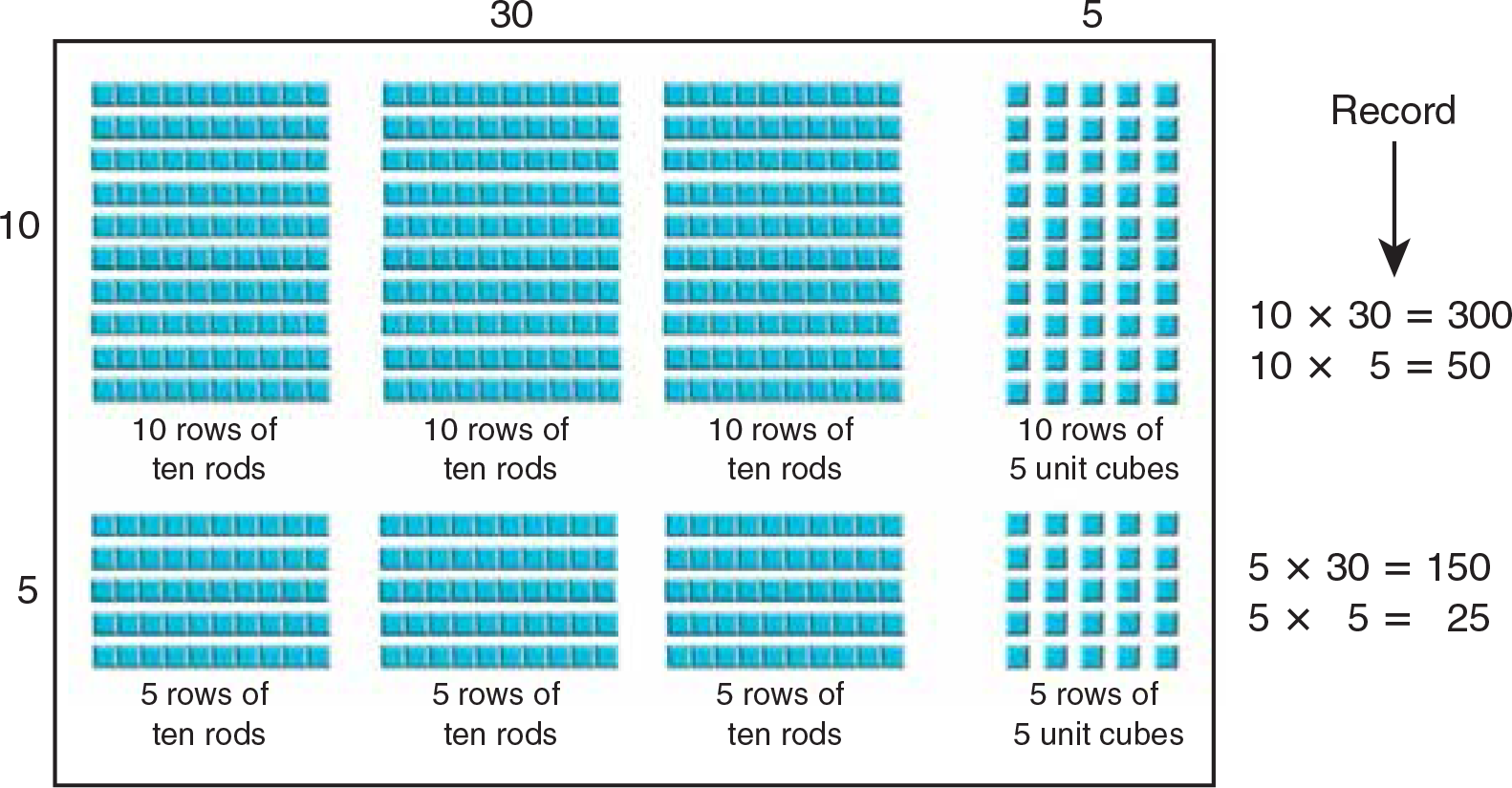An area model can be used to show the same multiplication: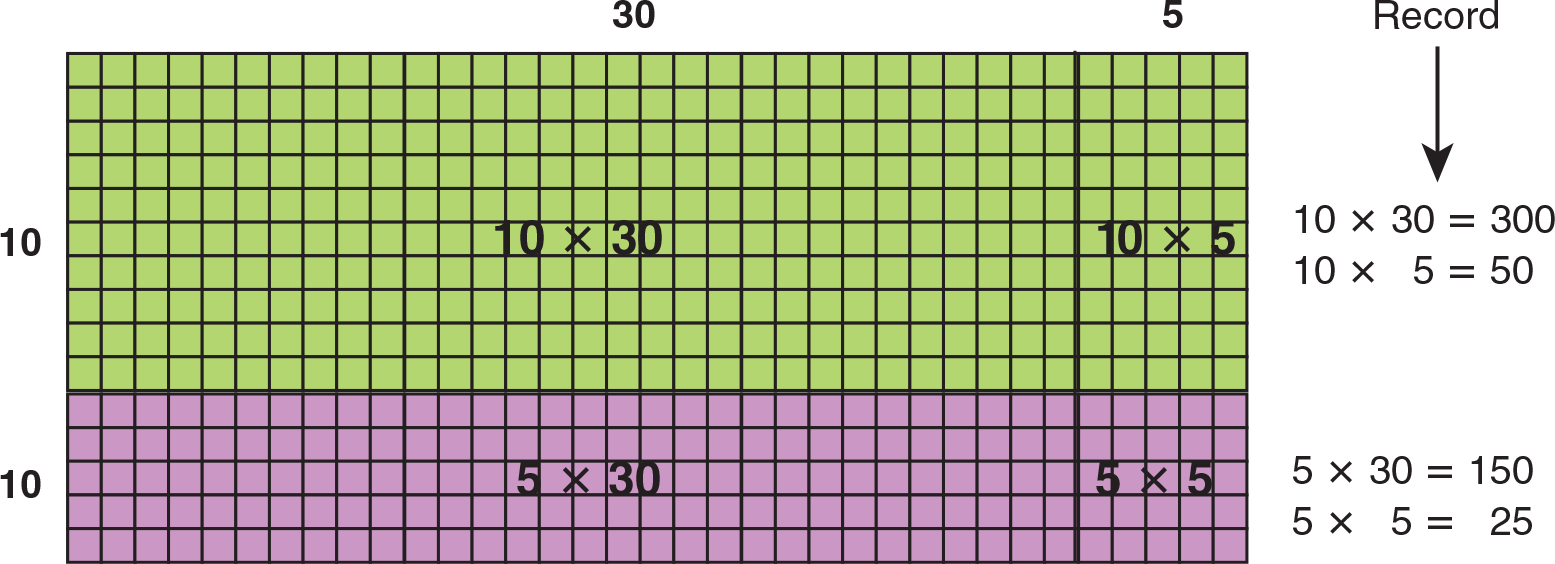As research demonstrates, students develop effective mathematical thinking when they understand the relationships among different representations of the same concept.1 Using the approaches above will allow students to see the relationships among concrete models, diagrams, and symbolic representations. Students will come to recognize that all three representations show the same product.

1 Goldin, Gerald and Nina Shiteingold. "Systems of Representation and the Development of Mathematical Concepts.” In The Roles of Representation in School Mathematics, 2001 Yearbook. Eds Albert A. Cuoco and Francis R. Curcio, National Council of Teachers of Mathematics. Reston, VA, 9.

## Referents to Estimate Length

### Throughout the elementary mathematics curriculum, estimation is presented as a precursor to finding actual results, and as a means for developing judgment about number, processes, and concepts. In measurement, estimation is developed in different ways, for example, as a referent against which to judge size, or as a procedure to help predict approximate answers.

To help students strengthen their measurement sense, have them choose and use referents to estimate length. (You can employ a similar activity for capacity and weight.)

Provide a collection of items familiar to students that they can use as referents.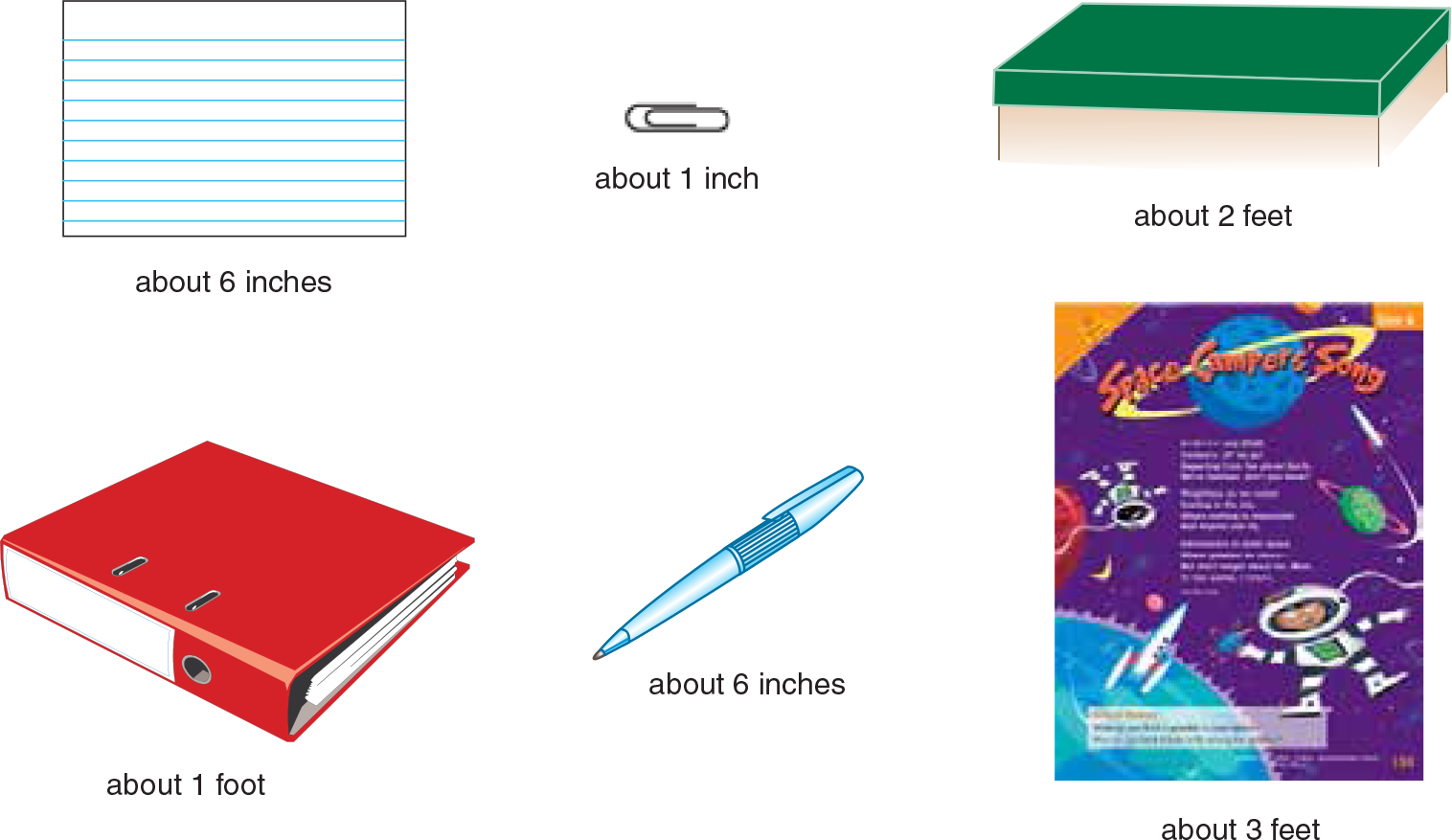Have students establish the approximate length of each referent. Then have them use the referents to estimate the lengths of objects or distances in the classroom. For example, they might see that the notebook spans about one third the distance across their desks. If one notebook length is about 1 foot, then the distance across the desk is about 3 feet.

Throughout the activity, it is important that students not view their estimates as right or wrong. Encourage students to refine their estimates by using referents to actually measure. Then have them use a ruler to measure. Students can compare their original estimate, the referent measure, and the actual measure to see how close the estimate is to the other.

To extend the activity, provide students with index cards and two-pocket folders. Have students name the most appropriate unit to measure the length of each item. Have students describe why they chose the unit they did.

References
Robert E. Reys, Mary M. Lindquist, Diana V. Lambdin, Nancy L. Smith, and Marilyn N. Suydam, Helping Children Learn Mathematics (New Jersey: John Wiley & Sons, 2004)

## Number Line

to Multiply and Divide Decimals

### In the previous chapter, students used decimal number lines for addition and subtraction. They have also encountered the number line as a support for multiplication and division of whole numbers.

Both verbal modeling and the number line can be used to reinforce the meaning of decimal multiplication and decimal division. Review the idea that a decimal can be rewritten as a fraction with a denominator of 10, 100, 1,000, and so on. This will reinforce for students the idea that a decimal number is always less than 1. Display a multiplication expression, such as 7 × 0.2, and guide the discussion as follows:

• How many groups are there? [7 groups]
• What will be in each group? [2 tenths, or 0.2]

As you demonstrate the multiplication on the number line, explain that you have drawn the line to 2.0 in intervals of 0.1. Guide students to recognize that the product will be greater than 1.0, but less than 2.0. Call on a volunteer to draw arrows over groups of two tenths, and then state the product, 1.4.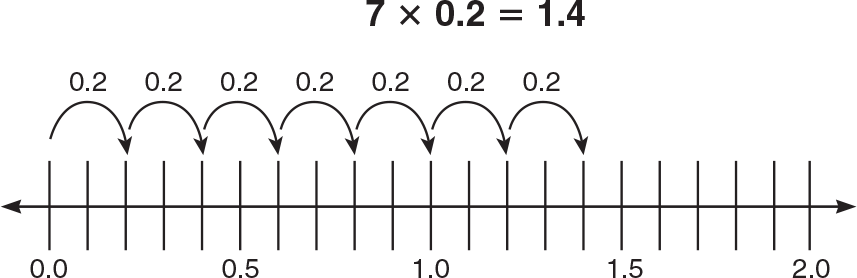Continue in a similar fashion to show division of decimals. Display the division expression 0.12 ÷ 0.04. Ask questions such as the following to get at the meaning of the expression:

• What is the dividend, or whole? [0.12, or 12 hundredths]
• Is the dividend greater than or less than 1? [less than 1]
• What do you need to find out in order to get the quotient? [How many groups of 0.04 are in 0.12?]

Draw the following number line, and, as before, call on a volunteer to draw arrows showing groups of 0.04. When students count the number of arrows, they can discover that the quotient is 3.